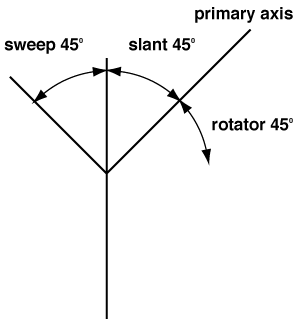# Arc attributes

• fme_geometry = fme_point
• fme_type = fme_arc
• fme_rotation: The rotation of the primary axis in degrees counterclockwise from the primary axis. If not set, then 0 is assumed.• fme_primary_axis: The length of the primary semi-axis (radius) of the defining ellipse measured in ground units.
• fme_secondary_axis: The length of the secondary semi-axis (radius) of the defining ellipse measured in ground units. For circular arcs this value is equal to the fme_primary_axis. This attribute is optional. If not present, it is assumed to be a circular arc with the same value as fme_primary_axis.
• fme_start_angle: Refer to Arcs (IFMEArc) for a detailed definition of `start_angle`.
• fme_sweep_angle:  Refer to Arcs (IFMEArc) for a detailed definition of `sweep_angle`.